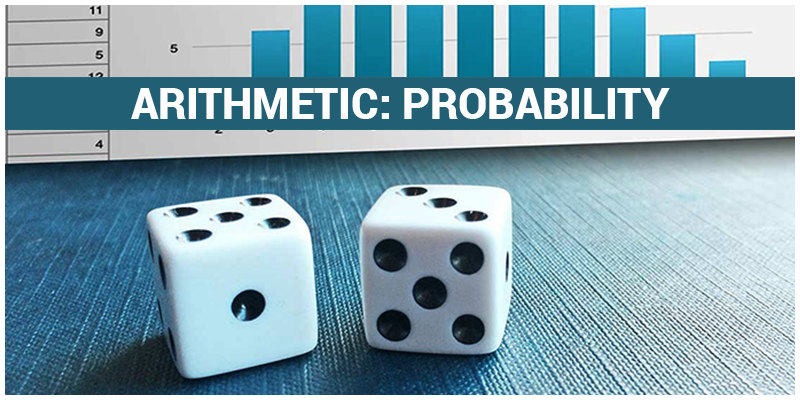# GMAT Quant: Arithmetic – ProbabilityProbability is the extent to which an event is likely to occur, measured by the ratio of the favourable cases to the whole number of cases possible.

When it comes to GMAT exam, all you have to do is make all the cases and select the favourable one.

$Probability = \frac{Number \; of \; Favourable \; Case}{Total \; Number \; of \; Cases}$

Points to Remember:

1. Whenever there is a word ‘and’, you should multiply the probability.
2. Whenever there is a word ‘or’, you should add the probability.
3. For mutually independent probability, you can add the probability.
4. $P(A\cup B) = P(A) + P(B) P(A\cap B)$

Let’s look at some of the problems –

1. There is 10% chance that it won’t snow during winter, there is a 20% chance that workplace will not be closed during winter. What is the probability that it will snow and workplace will be closed during the winter?

P(it will snow) = 1 -P (it won’t snow) =1 – 0.1 = 0.9

P(workplace will closed) = 1 – P (workplace will be open) = 1 – 0.2 =0.8

Since we can see the word ‘and’ we need to multiply the probability. Hence the probability is $0.8 \times 0.9 = 0.72 \; or \; 72%$

1. A telephone number contains 10 digits, including a 3- digit area code. Bob remembers the area code and next 4 digits of the number. He also remembers that the remaining digits are not 0, 2, 3, 5 or 9. If Bob tries to find the number by guessing the remaining digits at random, then find the probability that he will able to find the correct number in at most 2 attempts.
$P (Correct \; Attempt) = \frac{1}{5} \times \frac{1}{5} \times \frac{1}{5} = \frac{1}{125}$ $P (Incorrect \; Attempt) = 1 – \frac{1}{125} = \frac{124}{125}$ $P(Getting \; correct \; Number in 2 Attempts)$ $= P (First \; Attempt \; Correct) + P (First \; Attempt \; Incorrect \; and \; Second \; Attempt \; Correct)$ $= \frac{1}{125} + \frac{1}{125} \times \frac{124}{125} = \frac{249}{125^{2}}$

Read more: 10 Commandments to GMAT Verbal

We’ll be glad to help you in your GMAT preparation journey. You can ask for any assistance related to GMAT and MBA from us by calling us at +918884544444. You can write to us at gmat@byjus.com.